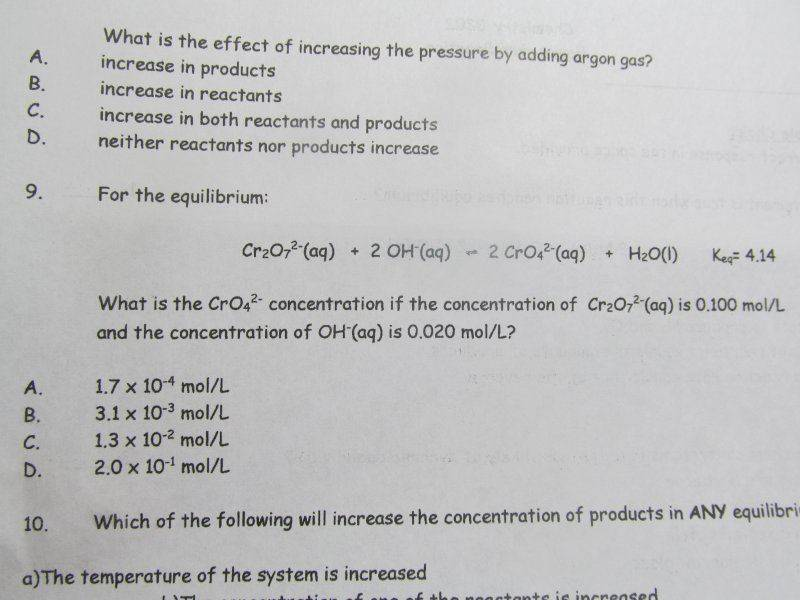# Concentration Problem

## Homework Statement

Question 9)## Homework Equations

$$K=\frac{[P]}{[R]}$$

## The Attempt at a Solution

What is confusing me is the wording. It merely says for the concentration of chromate. All this time we've been doing the equilibrium concentrations. Doing mole-mole with the Cr2O7 yields the answer to be D. I then assumed that it meant that they were the concentrations at equilibrium, which gave C to be the correct answer after preforming the equilibrium constant equation. Thanks for reading.

Borek
Mentor
Not sure what you mean by "doing mole-mole". I read the question as if 0.100 M was the equilibrium concentration, not the initial.

Not sure what you mean by "doing mole-mole". I read the question as if 0.100 M was the equilibrium concentration, not the initial.

Forgive me. Mole to mole ratio is what I meant to convey. Thank you for your input.

Borek
Mentor
In other words you tried to calculate equilibrium concentration following the stoichiometry?

Where did you got OH- from?

Quantum Defect
Homework Helper
Gold Member

## Homework Statement

Question 9)

View attachment 77944

## Homework Equations

$$K=\frac{[P]}{[R]}$$

## The Attempt at a Solution

What is confusing me is the wording. It merely says for the concentration of chromate. All this time we've been doing the equilibrium concentrations. Doing mole-mole with the Cr2O7 yields the answer to be D. I then assumed that it meant that they were the concentrations at equilibrium, which gave C to be the correct answer after preforming the equilibrium constant equation. Thanks for reading.

What is the expression for the equilibrium constant for this particular equilibrium?

True, it has [products] in the numerator and [reactants] in the denominator, but what do you do with the stoichiometric coefficients?

As Borek notes, they are giving you equilibrium values for the concentrations. If they weren't they would say something like "... the inital concentration of dichromate beofre equilibrium is reached is blah blah..."

As Borek notes, they are giving you equilibrium values for the concentrations. If they weren't they would say something like "... the inital concentration of dichromate beofre equilibrium is reached is blah blah..."

Yes. The issue was if they were equilibrium values or not. Usually it explicitly states.

Quantum Defect
Homework Helper
Gold Member
Yes. The issue was if they were equilibrium values or not. Usually it explicitly states.
What is the expression for the equilibrium constant, using concentrations? Where do the stoichiometric coefficients come in?